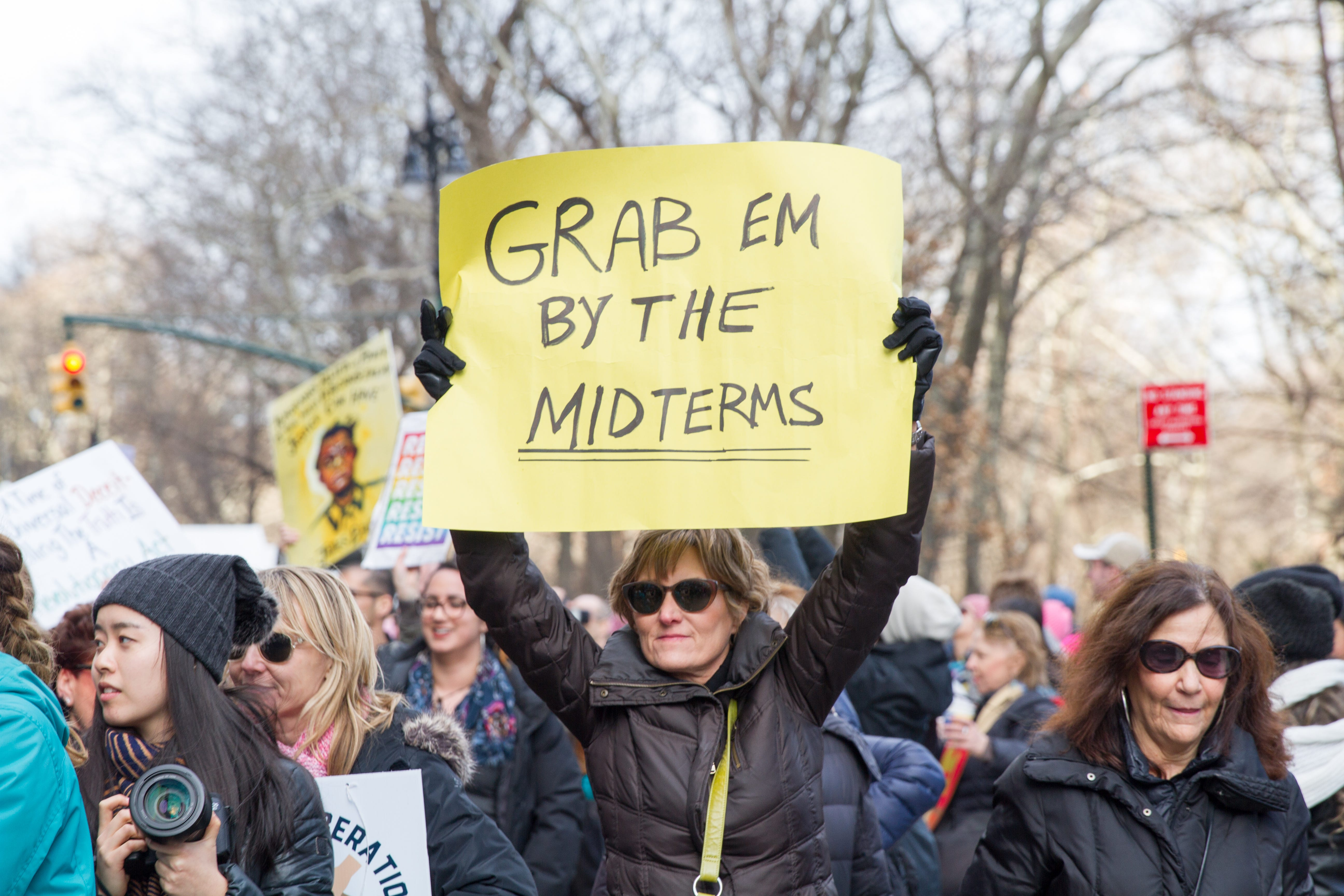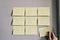# Using opinion polling technique for option pricing: StratumsPhoto by Mirah Curzer on Unsplash

One of the most used method used in opinion polls is dividing the sample for different stratums — can be done for different nationalities, age groups and etc. The reasoning is simple: when random polling is done within each group, experiments yield more accurate results with less number of observations(people) involved. Today, we will apply same technique to option pricing with Monte Carlo simulations in order to reduce computation time.

First, let’s start with a simple example : Calculate integral with Monte Carlo simulations (which is not a very appropriate usage of MC, but we will use it as an example).

But instead of using the integral directly, we can divide it in 2 or n.

But why did we do this? First of all, time complexity in monte carlo method is not linear. Doing 500 simulation 2 times cost less than 1000 simulation a time. Second, it improves accuracy, because sum of variances of subintegrals (stratums) is less than overall variance. Theoretically, if we divide the equation to more stratums, we get better and better results, but at some point, limits come into play.Photo by Kelly Sikkema on Unsplash

Implementation.

• Define meaningful stratums which are disjoint events that are relevant to the problem: divide the range of random variable into equal probability parts.
• Generate efficiently conditional probabilities of stratums
• Apply relevant function to each stratum (payoff funtion for stock prices)
• Sum up expected values of each stratums (conditional probabilites)

Find the Error of Estimate:

BONUS! How to divide into stratums? We can simply divide it to n equal parts, but there is a more effective way. Optimal distribution is if the number of values in each stratum can be computed by some constant C multiplied by probability of the stratum and standard deviation of that. Based on this, we need an additional function that generates n values untill n is bigger than the multiplication.

Practice.

Let’s use Black-Scholes model with constant volatility for predicting price of stock option:

Our standard generator can be defined as gen1:

`gen1 <- function(n){  return(S0*exp((r-D-sigma^2/2)*T+sigma*sqrt(T)*rnorm(n)))}`

The last multiplier, sigma*sqrt(T)*rnorm(n) identifies Brownian motion in the equation W(T).

Define our g function, which is simple payoff function for Call options

`g_call <- function(s){  return(exp(-r*T)*pmax(s-E,0))}`

Let’s suppose the option has strike E, with price at t=0 (now) is S0, expiration date is T, dividend yield D, mean rate of return r, and sigma volatility. We want to calculate the price of call option with error rate of epsilon, and (1-alpha) level of confidence.

`E=100S0=50T=1t=D=0r=0.1sigma=0.5epsilon=0.01alpha=0.05`

We used standard Monte Carlo method to calulate the option price before applying stratified sampling:

`answer1 <- MC2(g_call,gen1,epsilon,alpha = 0.05)#result: 1.902004e+00 3.795000e+06`

Computation gives us result1.9, as it is out-of money (OTM) option, with exercise price 100 while initial price is 50. This type of options are of interest, because with low price, if you can guess the final result, you can have better outcome. However, more interesting part for us is the second number: we need more then 3 million computation (n) in order to get the result with given precision.

Can we do better?

## Using stratums for Brownian Motion.

Instead of using arbitrary brownian motion, lets use stratums defined in between two limits. By limiting brownian motion, we limit the stock price, therefore, within each stratum the variance of stock price will be relatively smaller. We can easily check that variance within our previous generator was indeed high.

Instead of previous generator, I will create a new one, gen_strat, which applies stratified sampling to Brownian.

Important math: if we generate uniformly distributed values from 0 to 1, its quantile function is same with normal distributed values. But if we put restriction, such as subintervals instead of 0 to 1, we get conditional distributions.

`gen1_strat <- function(n,i,k){  BT <- sqrt(T)*qnorm(runif(n,(i-1)/k,i/k))#sqrt(T)*rnorm(n)  return(S0*exp((r-D-sigma^2/2)*T+sigma*BT))}`

If we generate 100 number with our new gen1_strat, from i=1 to k=1, it is logically same with non stratified sample.

But if divide it to 10 parts, and select the first, we will get numbers within the first quantile:

And by increasing k=2,3,4…10, the values of the stock price will increase, as it choses from corresponding quantile, with probability of each stratum 1/k. Let’s define our helper functions, and number of stratums, k

`#Generator for MC, gets n values, and feeds stratified generatorgen_strat <-function(n){  return (gen1_strat(n,i,k))}k=100 #number of stratumsresults <- rep(NA,k) #create space for result of each stratumni <- rep(NA,k) # create space for number of experiments#loop over number of stratums and run MCfor(i in 1:k){  tmp_result <- MC2(g_call,gen_strat,epsilon*sqrt(k),0.05)  results[i] <- tmp_result  ni[i] <- tmp_result }answer <- mean(results) #since p_i=i/k, final answern <- sum(ni) # final number of experiments neededcbind(answer,n)  [1,] 1.902807 467000`

If we run and get our answer for stratified sampling, we can see while expected value of stock price is same, the computation time decreased significantly. From 3.7 million to 467k. That’s a significant improvement!

## Conclusion.

Stratified sampling of Brownian motion can’t save you from all wrong decisions in life, but it will aid in monetary terms.

I talk to myself all the time.

## More from Lala Rustamli

I talk to myself all the time.